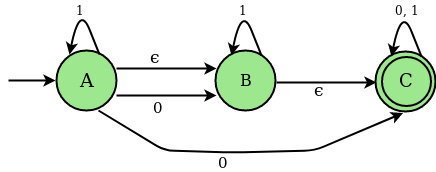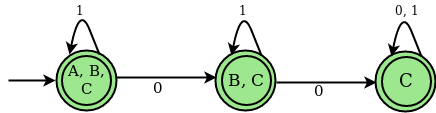Open in App
Not now

# Program to Implement NFA with epsilon move to DFA Conversion

• Difficulty Level : Hard
• Last Updated : 23 May, 2022

Non-deterministic Finite Automata (NFA) : NFA is a finite automaton where for some cases when a single input is given to a single state, the machine goes to more than 1 states, i.e. some of the moves cannot be uniquely determined by the present state and the present input symbol.

`An NFA can be represented as M = { Q, ∑, ∂, q0, F}`

Q → Finite non-empty set of states. ∑ → Finite non-empty set of input symbols. ∂ → Transitional Function. q0 → Beginning state. F → Final State

NFA with (null) or ∈ move : If any finite automata contains ε (null) move or transaction, then that finite automata is called NFA with ∈ moves

Example : Consider the following figure of NFA with ∈ move :Transition state table for the above NFA

Epsilon (∈) – closure : Epsilon closure for a given state X is a set of states which can be reached from the states X with only (null) or ε moves including the state X itself. In other words, ε-closure for a state can be obtained by union operation of the ε-closure of the states which can be reached from X with a single ε move in recursive manner. For the above example ∈ closure are as follows :

```∈ closure(A) : {A, B, C}
∈ closure(B) : {B, C}
∈ closure(C) : {C}```

Deterministic Finite Automata (DFA) : DFA is a finite automata where, for all cases, when a single input is given to a single state, the machine goes to a single state, i.e., all the moves of the machine can be uniquely determined by the present state and the present input symbol.

#### Steps to Convert NFA with ε-move to DFA :

Step 1 : Take ∈ closure for the beginning state of NFA as beginning state of DFA. Step 2 : Find the states that can be traversed from the present for each input symbol (union of transition value and their closures for each states of NFA present in current state of DFA). Step 3 : If any new state is found take it as current state and repeat step 2. Step 4 : Do repeat Step 2 and Step 3 until no new state present in DFA transition table. Step 5 : Mark the states of DFA which contains final state of NFA as final states of DFA.

Transition State Table for DFA corresponding to above NFA

DFA STATE DIAGRAMExamples :

```Input : 6
2
FC - BF
- C -
- - D
E A -
A - BF
- - -

Output :
STATES OF NFA :        A, B, C, D, E, F,

GIVEN SYMBOLS FOR NFA:     0, 1, eps

NFA STATE TRANSITION TABLE

STATES    |0    |1    eps
--------+------------------------------------
A    |FC     |-     |BF
B    |-     |C     |-
C    |-     |-     |D
D    |E     |A     |-
E    |A     |-     |BF
F    |-     |-     |-

e-Closure (A) :    ABF

e-Closure (B) :    B

e-Closure (C) :    CD

e-Closure (D) :    D

e-Closure (E) :    BEF

e-Closure (F) :    F

********************************************************

DFA TRANSITION STATE TABLE

STATES OF DFA :        ABF, CDF, CD, BEF,

GIVEN SYMBOLS FOR DFA:     0, 1,

STATES    |0    |1
--------+-----------------------
ABF    |CDF     |CD
CDF    |BEF     |ABF
CD    |BEF     |ABF
BEF    |ABF     |CD

Input :
9
2
- - BH
- - CE
D - -
- - G
- F -
- - G
- - BH
I - -
- -  -

Output :

STATES OF NFA :        A, B, C, D, E, F, G, H, I,

GIVEN SYMBOLS FOR NFA:     0, 1, eps

NFA STATE TRANSITION TABLE

STATES    |0    |1    eps
--------+------------------------------------
A    |-     |-     |BH
B    |-     |-     |CE
C    |D     |-     |-
D    |-     |-     |G
E    |-     |F     |-
F    |-     |-     |G
G    |-     |-     |BH
H    |I     |-     |-
I    |-     |-     |-

e-Closure (A) :    ABCEH

e-Closure (B) :    BCE

e-Closure (C) :    C

e-Closure (D) :    BCDEGH

e-Closure (E) :    E

e-Closure (F) :    BCEFGH

e-Closure (G) :    BCEGH

e-Closure (H) :    H

e-Closure (I) :    I

********************************************************

DFA TRANSITION STATE TABLE

STATES OF DFA :        ABCEH, BCDEGHI, BCEFGH,

GIVEN SYMBOLS FOR DFA:     0, 1,

STATES    |0    |1
--------+-----------------------
ABCEH    |BCDEGHI     |BCEFGH
BCDEGHI    |BCDEGHI     |BCEFGH
BCEFGH    |BCDEGHI     |BCEFGH     ```

Explanation : First line of the input contains the number of states (N) of NFA. Second line of the input says the number of input symbols (S). In example1 number of states of NFA is 6 i.e.( A, B, C, D, E, F) and 2 input symbols i.e. ( 0, 1). Since we are working on NFA with ∈ move, will be added as an extra input symbol. The next N lines contains the transition values for every state of NFA. The value of ith row, jth column indicates transition value for ith state on jth input symbol. Here in example1 transition(A, 0) : FC. Output contains the NFA, ∈ closure for every states of the corresponding NFA and DFA obtained by converting the input NFA. States and input symbols of the DFA are also specified. Below is the implementation of above approach :

## C

 `// C Program to illustrate how to convert e-nfa to DFA` `#include ``#include ``#include ``#define MAX_LEN 100` `char` `NFA_FILE[MAX_LEN];``char` `buffer[MAX_LEN];``int` `zz = 0;` `// Structure to store DFA states and their``// status ( i.e new entry or already present)``struct` `DFA {``  ``char` `*states;``  ``int` `count;``} dfa;` `int` `last_index = 0;``FILE` `*fp;``int` `symbols;` `/* reset the hash map*/``void` `reset(``int` `ar[], ``int` `size) {``  ``int` `i;` `  ``// reset all the values of``  ``// the mapping array to zero``  ``for` `(i = 0; i < size; i++) {``    ``ar[i] = 0;``  ``}``}` `// Check which States are present in the e-closure` `/* map the states of NFA to a hash set*/``void` `check(``int` `ar[], ``char` `S[]) {``  ``int` `i, j;` `  ``// To parse the individual states of NFA``  ``int` `len = ``strlen``(S);``  ``for` `(i = 0; i < len; i++) {` `    ``// Set hash map for the position``    ``// of the states which is found``    ``j = ((``int``)(S[i]) - 65);``    ``ar[j]++;``  ``}``}` `// To find new Closure States``void` `state(``int` `ar[], ``int` `size, ``char` `S[]) {``  ``int` `j, k = 0;` `  ``// Combine multiple states of NFA``  ``// to create new states of DFA``  ``for` `(j = 0; j < size; j++) {``    ``if` `(ar[j] != 0)``      ``S[k++] = (``char``)(65 + j);``  ``}` `  ``// mark the end of the state``  ``S[k] = ``'\0'``;``}` `// To pick the next closure from closure set``int` `closure(``int` `ar[], ``int` `size) {``  ``int` `i;` `  ``// check new closure is present or not``  ``for` `(i = 0; i < size; i++) {``    ``if` `(ar[i] == 1)``      ``return` `i;``  ``}``  ``return` `(100);``}` `// Check new DFA states can be``// entered in DFA table or not``int` `indexing(``struct` `DFA *dfa) {``  ``int` `i;` `  ``for` `(i = 0; i < last_index; i++) {``    ``if` `(dfa[i].count == 0)``      ``return` `1;``  ``}``  ``return` `-1;``}` `/* To Display epsilon closure*/``void` `Display_closure(``int` `states, ``int` `closure_ar[],``                     ``char` `*closure_table[],``                     ``char` `*NFA_TABLE[][symbols + 1],``                     ``char` `*DFA_TABLE[][symbols]) {``  ``int` `i;``  ``for` `(i = 0; i < states; i++) {``    ``reset(closure_ar, states);``    ``closure_ar[i] = 2;` `    ``// to neglect blank entry``    ``if` `(``strcmp``(&NFA_TABLE[i][symbols], "-") != 0) {` `      ``// copy the NFA transition state to buffer``      ``strcpy``(buffer, &NFA_TABLE[i][symbols]);``      ``check(closure_ar, buffer);``      ``int` `z = closure(closure_ar, states);` `      ``// till closure get completely saturated``      ``while` `(z != 100)``      ``{``        ``if` `(``strcmp``(&NFA_TABLE[z][symbols], "-") != 0) {``          ``strcpy``(buffer, &NFA_TABLE[z][symbols]);` `          ``// call the check function``          ``check(closure_ar, buffer);``        ``}``        ``closure_ar[z]++;``        ``z = closure(closure_ar, states);``      ``}``    ``}` `    ``// print the e closure for every states of NFA``    ``printf``("\n e-Closure (%c) :\t", (``char``)(65 + i));` `    ``bzero((``void` `*)buffer, MAX_LEN);``    ``state(closure_ar, states, buffer);``    ``strcpy``(&closure_table[i], buffer);``    ``printf``("%s\n", &closure_table[i]);``  ``}``}` `/* To check New States in DFA */``int` `new_states(``struct` `DFA *dfa, ``char` `S[]) {` `  ``int` `i;` `  ``// To check the current state is already``  ``// being used as a DFA state or not in``  ``// DFA transition table``  ``for` `(i = 0; i < last_index; i++) {``    ``if` `(``strcmp``(&dfa[i].states, S) == 0)``      ``return` `0;``  ``}` `  ``// push the new``  ``strcpy``(&dfa[last_index++].states, S);` `  ``// set the count for new states entered``  ``// to zero``  ``dfa[last_index - 1].count = 0;``  ``return` `1;``}` `// Transition function from NFA to DFA``// (generally union of closure operation )``void` `trans(``char` `S[], ``int` `M, ``char` `*clsr_t[], ``int` `st,``               ``char` `*NFT[][symbols + 1], ``char` `TB[]) {``  ``int` `len = ``strlen``(S);``  ``int` `i, j, k, g;``  ``int` `arr[st];``  ``int` `sz;``  ``reset(arr, st);``  ``char` `temp[MAX_LEN], temp2[MAX_LEN];``  ``char` `*buff;` `  ``// Transition function from NFA to DFA``  ``for` `(i = 0; i < len; i++) {` `    ``j = ((``int``)(S[i] - 65));``    ``strcpy``(temp, &NFT[j][M]);` `    ``if` `(``strcmp``(temp, "-") != 0) {``      ``sz = ``strlen``(temp);``      ``g = 0;` `      ``while` `(g < sz) {``        ``k = ((``int``)(temp[g] - 65));``        ``strcpy``(temp2, &clsr_t[k]);``        ``check(arr, temp2);``        ``g++;``      ``}``    ``}``  ``}` `  ``bzero((``void` `*)temp, MAX_LEN);``  ``state(arr, st, temp);``  ``if` `(temp != ``'\0'``) {``    ``strcpy``(TB, temp);``  ``} ``else``    ``strcpy``(TB, "-");``}` `/* Display DFA transition state table*/``void` `Display_DFA(``int` `last_index, ``struct` `DFA *dfa_states,``                 ``char` `*DFA_TABLE[][symbols]) {``  ``int` `i, j;``  ``printf``("\n\n********************************************************\n\n");``  ``printf``("\t\t DFA TRANSITION STATE TABLE \t\t \n\n");``  ``printf``("\n STATES OF DFA :\t\t");` `  ``for` `(i = 1; i < last_index; i++)``    ``printf``("%s, ", &dfa_states[i].states);``  ``printf``("\n");``  ``printf``("\n GIVEN SYMBOLS FOR DFA: \t");` `  ``for` `(i = 0; i < symbols; i++)``    ``printf``("%d, ", i);``  ``printf``("\n\n");``  ``printf``("STATES\t");` `  ``for` `(i = 0; i < symbols; i++)``    ``printf``("|%d\t", i);``  ``printf``("\n");` `  ``// display the DFA transition state table``  ``printf``("--------+-----------------------\n");``  ``for` `(i = 0; i < zz; i++) {``    ``printf``("%s\t", &dfa_states[i + 1].states);``    ``for` `(j = 0; j < symbols; j++) {``      ``printf``("|%s \t", &DFA_TABLE[i][j]);``    ``}``    ``printf``("\n");``  ``}``}` `// Driver Code``int` `main() {``  ``int` `i, j, states;``  ``char` `T_buf[MAX_LEN];` `  ``// creating an array dfa structures``  ``struct` `DFA *dfa_states = ``malloc``(MAX_LEN * (``sizeof``(dfa)));``  ``states = 6, symbols = 2;` `  ``printf``("\n STATES OF NFA :\t\t");``  ``for` `(i = 0; i < states; i++)` `    ``printf``("%c, ", (``char``)(65 + i));``  ``printf``("\n");``  ``printf``("\n GIVEN SYMBOLS FOR NFA: \t");` `  ``for` `(i = 0; i < symbols; i++)` `    ``printf``("%d, ", i);``  ``printf``("eps");``  ``printf``("\n\n");``  ``char` `*NFA_TABLE[states][symbols + 1];` `  ``// Hard coded input for NFA table``  ``char` `*DFA_TABLE[MAX_LEN][symbols];``  ``strcpy``(&NFA_TABLE, "FC");``  ``strcpy``(&NFA_TABLE, "-");``  ``strcpy``(&NFA_TABLE, "BF");``  ``strcpy``(&NFA_TABLE, "-");``  ``strcpy``(&NFA_TABLE, "C");``  ``strcpy``(&NFA_TABLE, "-");``  ``strcpy``(&NFA_TABLE, "-");``  ``strcpy``(&NFA_TABLE, "-");``  ``strcpy``(&NFA_TABLE, "D");``  ``strcpy``(&NFA_TABLE, "E");``  ``strcpy``(&NFA_TABLE, "A");``  ``strcpy``(&NFA_TABLE, "-");``  ``strcpy``(&NFA_TABLE, "A");``  ``strcpy``(&NFA_TABLE, "-");``  ``strcpy``(&NFA_TABLE, "BF");``  ``strcpy``(&NFA_TABLE, "-");``  ``strcpy``(&NFA_TABLE, "-");``  ``strcpy``(&NFA_TABLE, "-");``  ``printf``("\n NFA STATE TRANSITION TABLE \n\n\n");``  ``printf``("STATES\t");` `  ``for` `(i = 0; i < symbols; i++)``    ``printf``("|%d\t", i);``  ``printf``("eps\n");` `  ``// Displaying the matrix of NFA transition table``  ``printf``("--------+------------------------------------\n");``  ``for` `(i = 0; i < states; i++) {``    ``printf``("%c\t", (``char``)(65 + i));` `    ``for` `(j = 0; j <= symbols; j++) {``      ``printf``("|%s \t", &NFA_TABLE[i][j]);``    ``}``    ``printf``("\n");``  ``}``  ``int` `closure_ar[states];``  ``char` `*closure_table[states];` `  ``Display_closure(states, closure_ar, closure_table, NFA_TABLE, DFA_TABLE);``  ``strcpy``(&dfa_states[last_index++].states, "-");` `  ``dfa_states[last_index - 1].count = 1;``  ``bzero((``void` `*)buffer, MAX_LEN);` `  ``strcpy``(buffer, &closure_table);``  ``strcpy``(&dfa_states[last_index++].states, buffer);` `  ``int` `Sm = 1, ind = 1;``  ``int` `start_index = 1;` `  ``// Filling up the DFA table with transition values``  ``// Till new states can be entered in DFA table``  ``while` `(ind != -1) {``    ``dfa_states[start_index].count = 1;``    ``Sm = 0;``    ``for` `(i = 0; i < symbols; i++) {` `      ``trans(buffer, i, closure_table, states, NFA_TABLE, T_buf);` `      ``// storing the new DFA state in buffer``      ``strcpy``(&DFA_TABLE[zz][i], T_buf);` `      ``// parameter to control new states``      ``Sm = Sm + new_states(dfa_states, T_buf);``    ``}``    ``ind = indexing(dfa_states);``    ``if` `(ind != -1)``      ``strcpy``(buffer, &dfa_states[++start_index].states);``    ``zz++;``  ``}``  ``// display the DFA TABLE``  ``Display_DFA(last_index, dfa_states, DFA_TABLE);` `  ``return` `0;``}`

Use of NFA with ∈ move : If we want to construct an FA which accepts a language, sometimes it becomes very difficult or seems to be impossible to construct a direct NFA or DFA. But if NFA with ∈ moves is used, then the transitional diagram can be constructed and described easily.

My Personal Notes arrow_drop_up